# Jee Mains 24 February 2021 Shift-II Previous Year Paper

[tabs title=”JEE MAINS Previous Year Paper” type=”centered”]

[tab title=”PHYSICS”]

PHYSICS

SECTION A

Q 1: A body weighs 49 N on a spring balance at the north pole. What will be its weight recorded on the same weighing machine, if it is shifted to the equator?

[Use g = GM/R2 = 9.8 ms-2 and radius of earth, R = 6400 km.]

(A) 49 N

(B) 49.83 N

(C) 49.17 N

(D) 48.83 N

At North Pole, weight
Mg = 49
Now, at equator
g’ = g – ω2R
⇒ Mg’ = M(g – ω2R)
⇒ weight will be less than Mg at equator.
Alter:
g is maximum at the poles.
Hence from options only (D) has lesser value than 49N.

Q 2: The logic circuit shown below is equivalent to :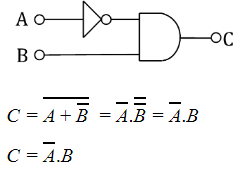Q 3: If one mole of an ideal gas at (P1, V1) is allowed to expand reversibly and isothermally (A to B) its pressure is reduced to one-half of the original pressure (see figure). This is followed by a constant volume cooling till its pressure is reduced to one-fourth of the initial value (B→C). Then it is restored to its initial state by a reversible adiabatic compression (C to A). The net work done by the gas is equal to:

(A) 0

(B) -RT/2( γ – 1)

(C) RT [ln2 -1/2( γ -1)]

(D) RT ln2

AB → Isothermal process
WAB = nRT ln2 = RT ln2
BC → Isochoric process
WBC = 0
WCA = P1V1 – (P1/4)X2V1)/(1 – γ)
= P1V1/2(1 – γ)
= RT/2(1 – γ )
WABCA = RT ln2 + RT/2(1 – γ)
= RT [ln2 – 1/2(γ – 1)]

Q 4: Match List – I with List – II.

Choose the correct answer from the options given below:

(A) (a)-(ii),(b)-(iv),(c)-(i),(d)-(iii)

(B) (a)-(vi),(b)-(iv),(c)-(i),(d)-(v)

(C) (a)-(ii),(b)-(iv),(c)-(vi),(d)-(iii)

(D) (a)-(vi),(b)-(v),(c)-(i),(d)-(iv)

(a) Source of microwave frequency – (ii) Magnetron
(b) Source of infrared frequency – (iv) Vibration of atom and molecules
(c) Source of gamma ray – (i) Radioactive decay of nucleus
(d) Source of X-ray – (iii) inner shell electron

Q 5: The period of oscillation of a simple pendulum is T = 2π√(L/g). Measured value of ‘L’ is 1.0 m from meter-scale having a minimum division of 1 mm and time of one complete oscillation is 1.95 s measured from stopwatch of 0.01 s resolution. The percentage error in the determination of ‘g’ will be:

(A) 1.33 %

(B) 1.30 %

(C) 1.13 %

(D) 1.03 %

T = 2π√(L/g)
T2 = 4𝜋2 [L/g]
g = 4𝜋2 [L/T2]
Δg/g = ΔL/L + 2ΔT/T
= [1mm/1m + 2(10×10-3)/1.95]×100
= 1.13 %

Q 6: When a particle executes SHM, the nature of graphical representation of velocity as a function of displacement is:

(A) elliptical

(B) parabolic

(C) straight line

(D) circular

We know that in SHM;
V = ω√(A2 – x2)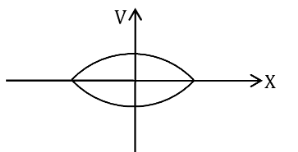Elliptical
Alternate:
x = A sinωt ⇒ sin ωt = x/A
v = Aω cos ωt ⇒ cos ωt = v/Aω
Hence (x/A)2 + (v/Aω)2 = 1
Which is the equation of an ellipse.

Q 7: In the given figure, a body of mass M is held between two massless springs, on a smooth inclined plane. The free ends of the springs are attached to firm supports. If each spring has spring constant k, the frequency of oscillation of given body is:

(A) (1/2π) √(2K/Mg sin α)

(B) (1/2π) √(K/Mg sin α)

(C) (1/2π) √(2K/M)

(D) (1/2π) √(K/2M)

Equivalent K = K + K = 2K
Now, T = 2π √(M/Keq)
⇒ T = 2π √(M/2K)
∴f = (1/2π) √(2K/M)

Q 8: Given below are two statements:

Statement I: PN junction diodes can be used to function as transistor, simply by connecting two diodes, back to back, which acts as the base terminal.

Statement II: In the study of transistor, the amplification factor β indicates ratio of the collector current to the base current.

In the light of the above statements, choose the correct answer from the options given below.

(A) Statement I is false but Statement II is true.

(B) Both Statement I and Statement II are true.

(C) Statement I is true but Statement II is false.

(D) Both Statement I and Statement II are false.

S-1
Statement 1 is false because in case of two discrete back to back connected diodes, there are four doped regions instead of three and there is nothing that resembles a thin base region between an emitter and a collector.
S-2
Statement-2 is true, as
β = IC/IB

Q 9: Figure shows a circuit that contains four identical resistors with resistance R = 2.0 Ω. Two identical inductors with inductance L = 2.0 mH and an ideal battery with emf E = 9.V. The current ‘i’ just after the switch ‘s’ is closed will be:

(A) 9A

(B) 3.0 A

(C) 2.25 A

(D) 3.37 A

Just when switch S is closed, inductor will behave like an infinite resistance. Hence, the circuit will be like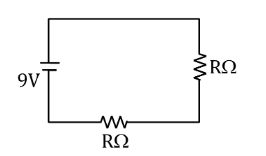Given: V = 9 V
From V = IR
I = V/R
Req. = 2 + 2 = 4 Ω
i = 9/4 = 2.25 A

Q 10: A circular hole of radius (a/2) is cut out of a circular disc of radius ‘a’ shown in figure. The centroid of the remaining circular portion with respect to point ‘O’ will be:

(A) (10/11)a

(B) (⅔)a

(C) (⅙)a

(D) (⅚)a

Let σ be the surface mass density of disc.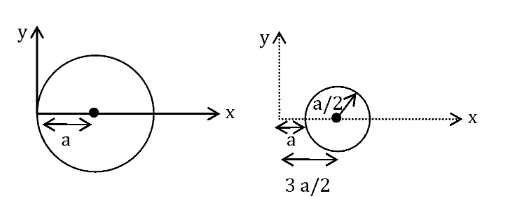Xcom= (m1x1 – m2x2)/(m1 – m2)
Where m = σπr2
Xcom= (σ×πa2×a) – (σπa2/4) × 3a/2)/(σπa2 – σπa2/4)
Xcom= (a – 3a/8)/(1 – ¼)
Xcom= (5a/8)/(¾)
Xcom= 5a/6

Q 11: The de Broglie wavelength of a proton and α-particle are equal. The ratio of their velocities is:

(A) 4:2

(B) 4:1

(C) 1:4

(D) 4:3

From De-Broglie’s wavelength:-
λ = h/mv
Given λP = λα
v ∝ 1/m
vp/vα = mα/mp = 4mp/mp
= 4/1

Q 12: On the basis of kinetic theory of gases, the gas exerts pressure because its molecules:

(A) suffer change in momentum when impinge on the walls of container.

(B) continuously stick to the walls of container.

(C) continuously lose their energy till it reaches wall.

(D) are attracted by the walls of container.

Based on kinetic theory of gases, molecules suffer change in momentum when impinge on the walls of container. Due to this they exert a force resulting in exerting pressure on the walls of the container.

Q 13: Two electrons each are fixed at a distance ‘2d’. A third charge proton placed at the midpoint is displaced slightly by a distance x (x<<d) perpendicular to the line joining the two fixed charges. Proton will execute simple harmonic motion having angular frequency:

(m = mass of charged particle)

(A) (q2/2πε0md3)1/2

(B) (πε0md3/2q2)1/2

(C) (2πε0md3/q2)1/2

(D) (2q2/πε0md3)1/2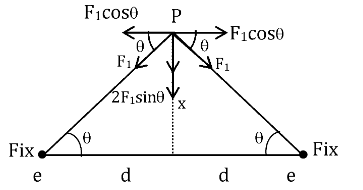Restoring force on proton:-
Fr = 2F1 sinθ where F1 = kq2/(d2 + x2)
Fr = 2Kq2x/[d2 + x2]3/2
x <<< d
Fr = 2kq2x/d3
= q2x/2πε0d3
= kx
K = q2/2πε0d3
Angular Frequency:-
ω = √(k/m)
ω = √(q2/2πε0md3)

Q 14: An X-ray tube is operated at 1.24 million volt. The shortest wavelength of the produced photon will be:

(A) 10-2 nm

(B) 10-3 nm

(C) 10-4 nm

(D) 10-1 nm

The minimum wavelength of photon will correspond to the maximum energy due to accelerating by V volts in the tube.
λmin = hc/eV
λmin = 1240nm-eV/1.24×106
λmin = 10-3 nm

Q 15: A soft ferromagnetic material is placed in an external magnetic fiel(D) The magnetic domains:

(A) decrease in size and changes orientation.

(B) may increase or decrease in size and change its orientation.

(C) increase in size but no change in orientation.

(D) have no relation with external magnetic field.

Atoms of ferromagnetic material in unmagnetized state form domains inside the ferromagnetic material. These domains have large magnetic moment of atoms. In the absence of magnetic field, these domains have magnetic moment in different directions. But when the magnetic field is applied, domains aligned in the direction of the field grow in size and those aligned in the direction opposite to the field reduce in size and also its orientation changes.

Q 16: According to Bohr atom model, in which of the following transitions will the frequency be maximum?

(A) n = 2 to n = 1

(B) n = 4 to n = 3

(C) n = 5 to n = 4

(D) n = 3 to n = 2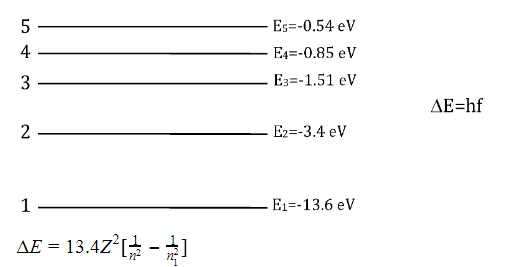Since, ΔE is maximum for the transition from n = 2 to n = 1
f is more for transition from n = 2 to n = 1.

Q 17: Which of the following equations represents a travelling wave?

(A) y = Aex^2 (vt + θ )

(B) y = A sin(15x – 2t)

(C) y = Aex cos(ωt – θ)

(D) y = A sin x cos ωt

Y = F(x, t)
For travelling wave y should be linear function of x and t and they must exist as (x ± vt)
Y = A sin (15x – 2t) which is a linear function in x and t.

Q 18: Zener breakdown occurs in a p-n junction having p and n both:

(A) lightly doped and have wide depletion layer.

(B) heavily doped and have narrow depletion layer.

(C) heavily doped and have wide depletion layer.

(D) lightly doped and have narrow depletion layer.

The zener breakdown occurs in the heavily doped p-n junction diode. Heavily doped p-n junction diodes have narrow depletion region. The narrow depletion layer width leads to a high electric field which causes the p-n junction breakdown.

Q 19: A particle is projected with velocity v0 along x-axis. A damping force is acting on the particle which is proportional to the square of the distance from the origin i.e. ma = -αx2. The distance at which the particle stops:

(A) (2v0/3α)1/3

(B) (3v02/2α)1/2

(C) (3v02/2α)1/3

(D) (2v02/3α)1/2

a = vdv/dx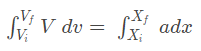Given:- vi = v0
Vf = 0
Xi = 0
Xf = x
From Damping Force: a = -αx2/m

-v02/2 = (-α/m) [x3/3]
x = [3mv02/2α]1/3
Most suitable answer could be (3) as mass ‘m’ is not given in any options.

Q 20: If the source of light used in a Young’s double slit experiment is changed from red to violet:

(A) the fringes will become brighter.

(B) consecutive fringe lines will come closer.

(C) the central bright fringe will become a dark fringe.

(D) the intensity of minima will increase.

β = λD/d
As λv<λR
βv< βR
⇒ Consecutive fringe lines will come closer.
⇒ (2)

Section – B

Q 1: A uniform thin bar of mass 6 kg and length 2.4 meter is bent to make an equilateral hexagon. The moment of inertia about an axis passing through the centre of mass and perpendicular to the plane of the hexagon is ______×10-1 kg m2.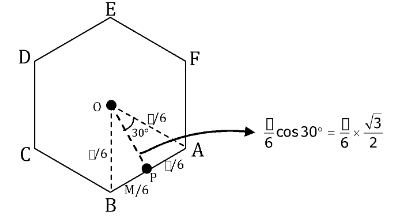MOI of AB about P : IABP = (M/6)(l/6)2/12= (6/100) [(24×24/12×36) + (24×24/36)×3/4]
= 0.8 kg m2
= 8×10-1 kg m2

Q 2: Two solids A and B of mass 1 kg and 2 kg respectively are moving with equal linear momentum. The ratio of their kinetic energies (K.E.)A : (K.E.)B will be A/1. So the value of A will be ________.

Given that, M1/M2 = 1/2
we know that
K = p2/2M
K1/K2 = (p2/2M1 )×2M2/p2
K1/K2 = M2/M1 = 2/1
A/1 = 2/1
⇒∴ A = 2

Q 3: An electromagnetic wave of frequency 3 GHz enters a dielectric medium of relative electric permittivity 2.25 from vacuum. The wavelength of this wave in that medium will be _______ ×10-2 cm.

εr = 2.25
Assuming non-magnetic material ⇒ μr = 1
Hence refractive index of the medium
n = √(μrεr )= √2.25 = 1.5
∴λvm=n
λm = C/f.n
= 3×108/3×109×1.5
= (⅔) 10-1 m
= 667×10-2 cm

Q 4: The root mean square speed of molecules of a given mass of a gas at 270C and 1 atmosphere pressure is 200 ms-1. The root mean square speed of molecules of the gas at 1270C and 2 atmosphere pressure is x/√3 ms-1. The value of x will be __________.

Vrms = √(3RT1/M0)
200 = √(3R×300/M0) ….(1)
Also, x/√3 = √(3R×400/M0) …(2)
(1)÷(2)
200/(x/√3) = √(300/400) = √(3/4)
⇒x = 400 m/s

Q 5: A signal of 0.1 kW is transmitted in a cable. The attenuation of cable is -5 dB per km and cable length is 20 km. The power received at the receiver is 10-xW. The value of x is ______.

[Gain in dB = 10 log10(P0/Pi)]

Power of signal transmitted: Pi = 0.1 Kw = 100w
Rate of attenuation = -5 dB/Km
Total length of path = 20 km
Total loss suffered = -5×20 = -100dB
Gain in dB = 10 log10(P0/Pi)
-100 = 10log10(P0/Pi)
log10(Pi/P0) = 10
log10(Pi/P0) = log101010
100/P0 = 1010
⇒ P0 = 1/108 = 10-8
⇒ x = 8

Q 6: A series LCR circuit is designed to resonate at an angular frequency ω0 = 105rad/s. The circuit draws 16W power from 120 V source at resonance. The value of resistance ‘R’ in the circuit is _______ Ω.

P = V2/R
16 = 1202/R
⇒ R = 14400/16
⇒ R = 900 Ω

Q 7: A uniform metallic wire is elongated by 0.04 m when subjected to a linear force F. The elongation, if its length and diameter is doubled and subjected to the same force, will be ________ cm.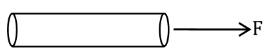y = Fl/A∆l
F/A = y∆l/l
F/A = y×0.04/l …(1)
When length & diameter is doubled.
⇒ F/4A = y × ∆l/2l …(2)
(1)÷(2)
(F/A)/(F/4A) = (y×0.04/l)y×∆l/2l
4 = 0.04×2/∆l
∆l = 0.02
∆l = 2×10-2
∴ x = 2

Q 8: A cylindrical wire of radius 0.5 mm and conductivity 5×107 S/m is subjected to an electric field of 10 mV/m. The expected value of current in the wire will be x3π mA. The value of x is ____.

We know that
J = σE
⇒ J = 5×107×10×10-3
⇒ J = 50×104 A/m2
Current flowing;
I = J × πR2
I = 50×104 ×π(0.5×10-3)2
I = 50×104×π×0.25×10-6
I = 125×10-3π
X = 5

Q 9: A point charge of +12 μC is at a distance 6 cm vertically above the centre of a square of side 12 cm as shown in figure. The magnitude of the electric flux through the square will be _____ ×103 Nm2/C.

Using Gauss law, it is a part of cube of side 12 cm and charge at centre,
ϕ = Q/6ε0= 12μc/6ε0

12×10−6 /6×8.85×10−12

= 226×103 Nm2/C

Q 10: Two cars are approaching each other at an equal speed of 7.2 km/hr. When they see each other, both blow horns having frequency of 676 Hz. The beat frequency heard by each driver will be ________ Hz. [Velocity of sound in air is 340 m/s.]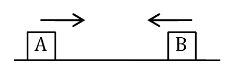Speed = 7.2 km/h = 2 m/s
Frequency as heard by A
f’A= fB(v + v0)/(v – vs)
f’A = 676(340 + 2)/(340 – 2)
f’A = 684Hz
∴ fBeat = f’A- fB
= 684 – 676
= 8 Hz

[/tab]

[tab title=”CHEMISTRY”]

Chemistry

SECTION A

Q 1. Given below are two statements:

(A) Both Statement I and Statement II are false

(B) Statement I is false but Statement II is true

(C) Statement I is true but Statement II is false

(D) Both Statement I and Statement II are true

For survival of aquatic life dissolved oxygen is responsible for its optimum limit 6.5 ppm and optimum limit of BOD ranges from 10-20 ppm & BOD stands for biochemical oxygen demand.

Q 2. Which one of the following carbonyl compounds cannot be prepared by addition of water on an alkyne in the presence of HgSO4 and H2SO4?

Reaction of Alkyne with HgSO4 & H2SO4 follow as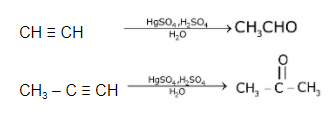Hence, by this process preparation of CH3CH2CHO can’t be possible.

Q 3. Which one of the following compounds is non-aromatic?(Not planar)
Hence, it is non-aromatic.

Q 4. The incorrect statement among the following is:

(A) VOSO4 is a reducing agent

(B) Red color of ruby is due to the presence of CO3+

(C) Cr2O3 is an amphoteric oxide

(D) RuO4 is an oxidizing agent

Red color of ruby is due to presence of CrO3 or Cr+6 not CO3+

Q 5. According to Bohr’s atomic theory:

(a) Kinetic energy of electron is ∝ Z2 / n2

(b) The product of velocity (v) of electron and principal quantum number (n). ‘vn’ ∝ z2

(c) Frequency of revolution of electron in an orbit is ∝ Z3 / n3

(d) Coulombic force of attraction on the electron is ∝ Z3 / n4

Choose the most appropriate Answer from the options given below

(A) (C) only

(B) (A) and (D) only

(C) (A) only

(D) (A), (C) and (D) only

(a) KE = –TE = 13.6 × Z2 / n2 eV
KE α Z2 / n2
(b) V = 2.188 × 106 × z / n m/s
So, Vn ∝ Z
Frequency = V / 2πr
F α Z2 / n2 [∴ r α Z2 / n2 and v α Z / n ]
(d) Force ∝ Z2 / r2
So, F ∝ Z3 / n4
So, only statement (A) is correct.

Q 6. Match List-I with List-II

(A) (A)-(iv), (B)-(iii), (C)-(ii), (D)-(i)

(B) (A)-(i), (B)-(iii), (C)-(iv), (D)-(ii)

(C) (A)-(ii), (B)-(iv), (C)-(iii), (D)-(i)

(D) (A)-(iv), (B)-(iii), (C)-(i), (D)-(ii)

(a) Valium (iv) Tranquilizer
(b) Morphine (iii) Analgesic
(c) Norethindrone (i) Antifertility drug
(d) Vitamin B12 (ii) Pernicious anemia

Q 7. Match List-I with List-II

Choose the correct Answer from the options given below:

(A) (A)-(ii), (B)-(i), (C)-(iv), (D)-(iii)

(B) (A)-(ii), (B)-(iv), (C)-(iii), (D)-(i)

(C) (A)-(iv), (B)-(ii), (C)-(iii), (D)-(i)

(D) (A)-(i), (B)-(iv), (C)-(ii), (D)-(iii)

Range of visible region: 390 nm – 760 nm
VIBGYOR
Violet – Red
LiCl Crimson Red
NaCl Golden yellow
RbCl Violet
CsCl Blue
So, LiCl which is crimson have wavelengths close to red in the spectrum of visible region which is as per given data.

Q 8. Match List-I and List-II.

(A) (A)-(ii), (B)-(i), (C)-(iv), (D)-(iii)

(B) (A)-(iii), (B)-(iv), (C)-(i), (D)-(ii)

(C) (A)-(iii), (B)-(i), (C)-(iv), (D)-(ii)

(D) (A)-(ii), (B)-(iv), (C)-(i), (D)-(iii)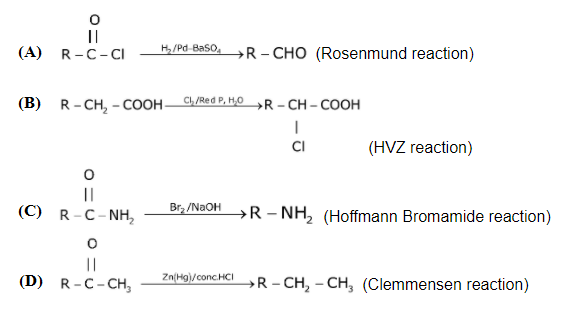Q 9. In polymer Buna-S: ‘S’ stands for

(A) Styrene

(B) Sulphur

(C) Strength

(D) Sulphonation

Buna-S is the co-polymer of buta-1,3-diene & styrene

Q 10. Most suitable salt which can be used for efficient clotting of blood will be:

(A) Mg(HCO3)2

(B) FeSO4

(C) NaHCO3

(D) FeCl3

Blood is a negative sol, according to Hardy-Schulz’s rule, the cation with high charge has high coagulation power. Hence, FeCl3 can be used for clotting blood.

Q 11. Given below are two statements: one is labelled as Assertion A and the other is labelled as Reason R.

Assertion A: Hydrogen is the most abundant element in the Universe, but it is not the most abundant gas in the troposphere.

Reason R: Hydrogen is the lightest element.

In the light of the above statements, choose the correct Answer from the given below

(1) A is false but R is true

(2) Both A and R are true and R is the correct explanation of A

(3) A is true but R is false

(4) Both A and R are true but R is NOT the correct explanation of A

(A) A is false but R is true

(B) Both A and R are true and R is the correct explanation of A

(C) A is true but R is false

(D) Both A and R are true but R is NOT the correct explanation of A

Hydrogen is the most abundant element in the universe because all luminous bodies of the universe i.e. stars and nebulae are made up of hydrogen which acts as nuclear fuel and fusion reaction is responsible for their light.

Q 12. What is the correct sequence of reagents used for converting nitrobenzene into m- dibromobenzene?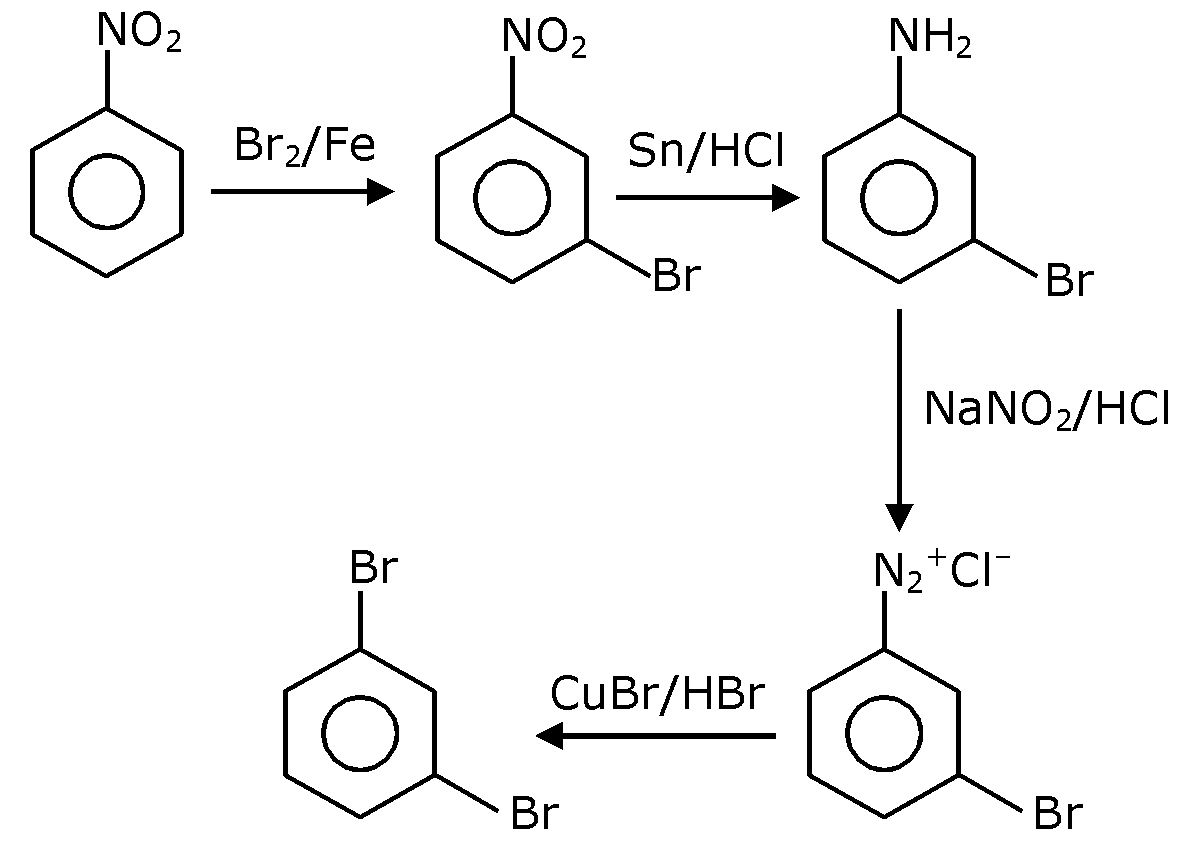Q 13. The correct shape and I-I-I bond angles respectively in I3 ion are:

(A) Trigonal planar; 120o

(B) Distorted trigonal planar; 135o and 90o

(C) Linear; 180º

(D) T-shaped; 180º and 90º

I3 has sp3d hybridization (2 BP + 3 LP) and linear geometry.

Q 14. What is the correct order of the following elements with respect to their density?

(A) Cr < Fe < Co < Cu < Zn

(B) Cr < Zn < Co < Cu < Fe

(C) Zn < Cu < Co < Fe < Cr

(D) Zn < Cr < Fe < Co < Cu

Fact Based
Density depends on many factors like atomic mass. atomic radius and packing efficiency.

Q 15. The Correct set from the following in which both pairs are in correct order of melting point is

(A) LiF > LiCl ; NaCl > MgO

(B) LiF > LiCl ; MgO > NaCl

(C) LiCl > LiF ; NaCl > MgO

(D) LiCl > LiF ; MgO > NaCl

Generally,
M.P. ∝ Lattice energy = KQ1Q2 / r+ + r

∝ (packing efficiency)

Q 16. The calculated magnetic moments (spin only value) for species

[FeCl4]2–, [Co(C2O4)3]3– and MnO2–4 respectively are:

[FeCl4]2–
Fe2+ 3d6 → 4 unpaired electrons. as Cl in a weak field liquid.

Q 17. Which of the following reagent is suitable for the preparation of the product in the following reaction?

(A) Red P + Cl2

(B) NH2-NH2/ C2H5ONa+

(C) Ni/H2

(D) NaBH4It is a wolf-kishner reduction of carbonyl compounds.

Q 18. The diazonium salt of which of the following compounds will form a coloured dye on reaction with β-Naphthol in NaOH?Orange bright dye.

Q 19. The correct order of the following compounds showing increasing tendency towards nucleophilic substitution reaction is:

(A) (iv) < (i) < (iii) < (ii)

(B) (iv) < (i) < (ii) < (iii)

(C) (i) < (ii) < (iii) < (iv)

(D) (iv) < (iii) < (ii) < (i)Reactivity ∝ – M group present at o/p position.

Q 20. Match List-I with List-II

(A) (A)-(iv), (B)-(iii), (C)-(ii), (D)-(i)

(B) (A)-(i), (B)-(ii), (C)-(iii), (D)-(iv)

(C) (A)-(iii), (B)-(i), (C)-(iv), (D)-(ii)

(D) (A)-(ii), (B)-(iv), (C)-(i), (D)-(iii)

Siderite FeCO3
Calamine ZnCO3
Kaolinite Si2Al2O5(OH)4 or Al2O3.2SiO2.2H2O
Malachite CuCO3.Cu(OH)2

Section B

Q 1. The formula of a gaseous hydrocarbon which requires 6 times of its own volume of O2 for complete oxidation and produces 4 times its own volume of CO2 is CxHy. The value of y is

CxHy + 6O2 4CO2 + y/2 H2O
Applying POAC on ‘O’ atoms
6 × 2 = 4 × 2 + y/2 × 1
y/2 = 4 ⇒ y = 8

Q 2. The volume occupied by 4.75 g of acetylene gas at 50°C and 740 mmHg pressure is _______L. (Rounded off to the nearest integer)

(Given R = 0.0826 L atm K–1 mol–1)

T = 50C = 323.15 K
P = 740 mm of Hg = 740 / 760 atm
V = ?
moles (n) = 4.75 / 26 atm
V = 4.75 / 26 × 0.0821 × 323.15 / 740 × 760
V = 4.97 5 Lit

Q 3. Sucrose hydrolysis in acid solution into glucose and fructose following first order rate law with a half-life of 3.33 h at 25ºC. After 9h, the fraction of sucrose remaining is f. The value of is _________× 10–2 (Rounded off to the nearest integer)

[Assume: ln10 = 2.303, ln2 = 0.693]

Sucrose  __(Hydrolysis)→  Glucose + Fructose
t1/2 = 3.33h = 10 / 3h

Ct = C0 / {2 × T/t1/2}

Fraction of sucrose remaining = f = Ct/Co = 1/ {2 × T/t1/2}

1/f = 2^(t/t1/2)

Log(1/f) = log(2^(t/t1/2)) = t/t1/2 log(2)

9/ (10/3) × 0.3 – 8.1/10 – 0.81

= x × 10–2

x = 81

Q 4. The total number of amines among the following which can be synthesized by Gabriel synthesis is _______

Only 1o amines can be prepared by Gabriel synthesis.

Q 5. 1.86 g of aniline completely reacts to form acetanilide. 10% of the product is lost during purification. Amount of acetanilide obtained after purification (in g) is ____× 10–2.93 g Aniline produce 135 g acetanilide
1.86 g produce 135 × 1.86 / 93 = 2.70 g
At 10% loss, 90% product will be formed after purification.

Q 6. Among the following allotropic forms of sulphur, the number of allotropic forms, which will show paramagnetism is ______.

(a) α-sulphur (B) β-sulphur (C) S2-form

S2 is like O2 i.e. paramagnetic as per molecular orbital theory.

Q 7. C6H6 freezes at 5.5ºC. The temperature at which a solution of 10 g of C4H10 in 200 g of C6H6 freeze is ________ C. (The molal freezing point depression constant of C6H6 is 5.12C/m).

ΔTf = i × Kf × m
= 1 × 5.12 × 10 / 58200 × 1000
∆Tf = 5.12 × 50 / 58 = 4.414
Tf(solution) = Tk(solvent) – ΔT = 5.5 – 4.414 = 1.086oC
≈ 1.09°C = 1 (nearest integer)

Q 8. Assuming ideal behavior, the magnitude of log K for the following reaction at 25ºC is x × 10–1. The value of x is __________. (Integer Answer)

3HC ≡ CH(g) ⇌ C6H6 (l)

[Given: ΔfG° (HC = CH) = – 2.04 × 105] mol–1

ΔfG°(C6H6) = – 1.24 × 105 J mol–1;

R = 8.314 J K–1 mol–1

ΔG°r = ΔG°f [C6H6 (l)] – 3 × ΔG°f [HC = CH]

Q 9. The magnitude of the change in oxidising power of the MnO4-/ Mn2+ couple is x × 10–4 V, if the H+ concentration is decreased from 1M to 10–4 M at 25°C. (Assume concentration of MnO4- and Mn2+ to be same on change in H+ concentration). The value of x is _____. (Rounded off to the nearest integer).

[Given: 230RT / F = 0.059]

5e- + MnO4 + 8H → Mn+2 + 4H2O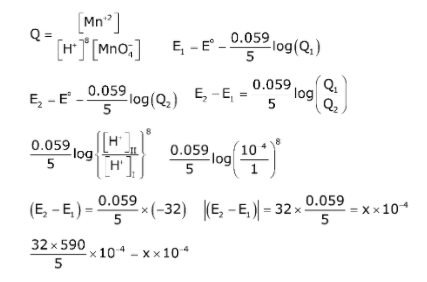= 3776 × 10–4
So, x = 3776

Q 10. The solubility product of PbI2 is 8.0 × 10–9. The solubility of lead iodide in 0.1 molar solution of lead nitrate is x × 10–6 mol/L. The value of x is ________ (Rounded off to the nearest integer)

Given: √2 = 1.41

PbI2(s) ⇌ Pb2+ (aq) + 2I (aq)
S+0.1 2s
KSP (PbI2) = 8 × 109
KSP = [Pb+2][I]2
8 × 10–9 = (S + 0.1) (2S)2 ⇒ (8 × 10–9 + 0.1) × 4S2
⇒ S2 = 2 × 10–8
S = 1.414 × 10–4 mol/Lit
= x × 10–6 mol/Lit
∴ x = 141.4 141

[/tab]

[tab title=”MATHS”]

Maths

SECTION A

Q 1: Let a, b, c be in arithmetic progression. Let the centroid of the triangle with vertices (a, c), (2, b) and (a, b) be (10/3, 7/3). If α, β are the roots of the equation ax2 + bx + 1 = 0, then the value of α2 + β2 – αβ is:

(A) 71/256

(B) -69/256

(C) 69/256

(D) -71/256

2b = a + c
(2a + 2)/3 = 10/3 and (2b + c)/3 = 7/3
⇒ a = 4
2b + c = 7, 2b – c = 4 },solving
b = 11/4 and c = 3/2
∴ Quadratic equation is 4x2+ (11/4)x + 1 = 0
∴ The value of (α + β)2 – 3αβ = (121/256) – (¾)
= -71/256

Q 2: The value of the integral,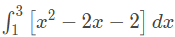where [x] denotes the greatest integer less than or equal to x , is :

(A) –4

(B) –5

(C) -√2 – √3 – 1

(D) -√2 – √3 + 1

I =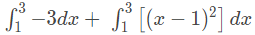Put x – 1 = t ; dx = dt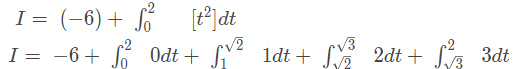I = -6 + (√2 – 1) + 2√3 – 2√2 + 6 – 3√3
I = -1 – √2 – √3

Q 3: Let f: R → R be defined as

Let A = {x ∈ R ∶ f is increasing}. Then A is equal to :

(A) (-5, -4) ∪ (4,∞)

(B) (-5, ∞)

(C) (-∞, -5) ∪ (4, ∞)

(D) (-∞, -5) ∪ (-4, ∞)

f’(x) = {-55 ; x< -5 6(x2 – x – 20) ; -5 < x < 4 6(x2– x – 6) ; x > 4
⇒ f’(x) = {-55 ; x< -5 6(x – 5)(x + 4) ; –5 < x < 4 6(x – 3)(x + 2) ; x > 4
Hence, f(x) is monotonically increasing in (-5, -4) ∪ (4, ∞)

Q 4: If the curve y = ax2 + bx + c,x ∈ R passes through the point (1, 2) and the tangent line to this curve at origin is y = x, then the possible values of a, b, c are:

(A) a = 1, b = 1, c = 0

(B) a = -1, b = 1, c = 1

(C) a = 1, b = 0, c = 1

(D) a = 1/2, b = 1/2 ,c = 1

2 = a + b + c
dy/dx = 2ax + b, (dy/dx)(0,0) = 1
⇒ b = 1 and a + c = 1
Since (0, 0) lies on curve,
∴ c = 0, a = 1
TRICK: (0, 0) lies on the curve. Only option (1) has c = 0

Q 5: The probability that two randomly selected subsets of the set {1, 2, 3, 4, 5} have exactly two elements in their intersection, is:

(A) 65/27

(B) 135/29

(C) 65/28

(D) 35/27

Let A and B be two subsets.
For each x∈{1,2,3,4,5} , there are four possibilities:
x ∈ A∩B, x∈ A’∩B, x ∈ A∩B’, x ∈ A’∩B’
So, the number of elements in sample space =45
Required probability
= (5C2 × 33)/45
= (10×27)/210
= 135/29

Q 6: The vector equation of the plane passing through the intersection of the planes

and

and the point (1, 0, 2) is:

Family of planes passing through the intersection of planes is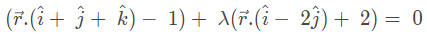The above curve passes through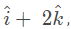(3 − 1) + λ(1 + 2) = 0
⇒ λ = -2/3
Hence, equation of plane is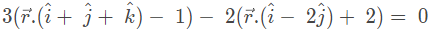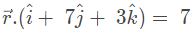TRICK: Only option (2) satisfies the point (1, 0, 2)

Q 7: If P is a point on the parabola y = x2 + 4 which is closest to the straight line y = 4x − 1, then the coordinates of P are :

(A) (–2, 8)

(B) (1, 5)

(C) (3, 13)

(D) (2, 8)

Tangent at P is parallel to the given line.
dy/dx|P = 4
⇒2x1 = 4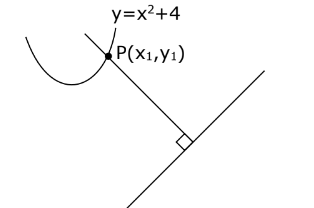⇒x1 = 2
Required point is (2, 8)

Q 8: Let A and B be 3×3 real matrices such that A is symmetric matrix and B is skew-symmetric matrix. Then the system of linear equations (A2B2 − B2A2)X = O, where X is a 3×1 column matrix of unknown variables and O is a 3×1 null matrix, has:

(A) a unique solution

(B) exactly two solutions

(C) infinitely many solutions

(D) no solution

AT = A, BT = –B
Let A2B2 − B2A2= P
PT = (A2B2 – B2A2)T
= (A2B2)T – (B2A2)T
= (B2)T (A2)T– (A2)T (B2)T
= B2A2 – A2B
⇒ P is a skew-symmetric matrix.

∴ ay + bz = 0 …(1)
–ax + cz = 0 …(2)
–bx – cy =0 …(3)
From equation (1), (2), (3)
Δ = 0 and Δ1 = Δ2= Δ3 = 0
∴ System of equations has infinite number of solutions.

Q 9: If n ≥ 2 is a positive integer, then the sum of the series n+1C2 + 2(2C2 + 3C2 + 4C2 + …. + nC2) is

(A) n(n + 1)2 (n + 2)/12

(B) n(n – 1)(2n + 1)/6

(C) n(n + 1)(2n + 1)/6

(D) n(2n + 1)(3n + 1)/6

2C2 = 3C3
Let S = 3C3 + 3C2 + ……. + nC2 = n+1C3 (∵ nCr + nCr–1 = n+1Cr)
n+1C2 + n+1C3 + n+1C3
= n+2C2 + n+1C3
=(n + 2)!/3!(n – 1)! + (n + 1)!/3!(n – 2)!
=(n + 2)(n + 1)n/6 + (n + 1)(n)(n – 1)/6 = (n(n + 1)(2 + 1))/6
TRICK : Put n = 2 and verify the options.

Q 10: If a curve y = f(x) passes through the point (1, 2) and satisfies xdy/dx + y = bx4, then for what value of b,

(A) 5

(B) 62/5

(C) 31/5

(D) 10

dy/dx + y/x = bx3
I.F. =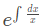= x
∴ yx = ∫bx4 dx = bx5/5 + c
The above curve passes through (1, 2).
2 = b/5 + c
Also,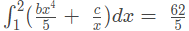⇒ (b/25) ×32 + c ln 2 – b/25 = 62/5
⇒ c = 0 and b = 10

Q 11: The area of the region: R{(x, y): 5x2 ≤ y ≤ 2x2 + 9} is:

(A) 9√3 square units

(B) 12√3 square units

(C) 11√3 square units

(D) 6√3 square units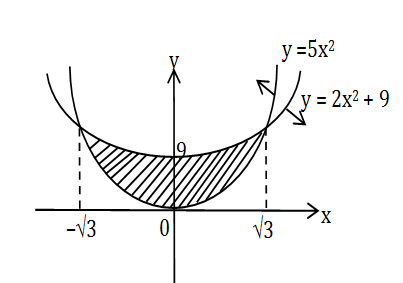Required area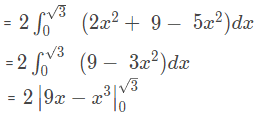= 12√3

Q 12: Let f(x) be a differentiable function defined on [0,2] such that f’(x) =f ‘(2 – x) for all x∈(0, 2), f(0) = 1 and f(2) = e2. Then the value of is:

(A) 1 + e2

(B) 1 – e2

(C) 2(1 – e2)

(D) 2(1+e2)

f'(x) = f'(2 – x)
On integrating both sides, we get
f(x) = -f(2 – x)+c
Put x = 0
f(0) + f(2) = c
⇒ c = 1+ e2
⇒ f(x) + f(2 – x) = 1 + e2
I =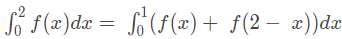= 1+e2

Q 13: The negation of the statement ~p∧(p∨q) is∶

(A) ~p∧q

(B) p∧~q

(C) ~p∨q

(D) p∨∼q

Negation of ~p∧(p∨q) is
∼[~p∧(p∨q)]
≡ p∨ ∼(p∨q)
≡ p∨(∼p∧∼q)
≡ (p∨∼p)∧(p∨∼q)
≡ T∧(p∨∼q), where T is tautology.
≡ p∨∼q

Q 14: For the system of linear equations: x − 2y = 1, x − y + kz = −2, ky + 4z = 6,k ∈ R

Consider the following statements:

(A) The system has unique solution if k ≠ 2,k ≠ −2.

(B) The system has unique solution if k = -2.

(C) The system has unique solution if k = 2.

(D) The system has no-solution if k = 2.

(E) The system has infinite number of solutions if k ≠ -2.

Which of the following statements are correct?

(A) (B) and (E) only

(B) (C) and (D) only

(C) (A) and (D) only

(D) (A) and (E) only

x -2y + 0.z = 1
x – y + kz = -2
0.x + ky + 4z = 6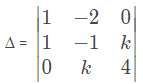= 4 – k2
For unique solution, 4 – k2 ≠ 0
k ≠ ±2
For k = 2,
x – 2y + 0.z =1
x – y + 2z = -2
0.x + 2y + 4z = 6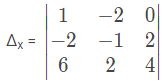= -8 + 2 (-20)
⇒Δx= -48 ≠ 0
For k = 2,Δx ≠ 0
So, for k = 2, the system has no solution.

Q 15: For which of the following curves, the line x + √3y = 2√3 is the tangent at the point (3√3/2, 1/2)?

(A) x2 + 9y2 = 9

(B) 2x2 – 18y2 = 9

(C) y2 = x/(6√3)

(D) x2 + y2 = 7

Tangent to x2 + 9y2 = 9 at point (3√3/2, 1/2) is x(3√3)/2 + 9y(1/2) = 9
⇒3√3 x + 9y = 18
⇒x +√3 y = 2√3
⇒ Option (A) is true.

Q 16: The angle of elevation of a jet plane from a point A on the ground is 600. After a flight of 20 seconds at the speed of 432 km/hour, the angle of elevation changes to 300. If the jet plane is flying at a constant height, then its height is:

(A) 1200√3 m

(B) 1800√3 m

(C) 3600√3 m

(D) 2400√3 m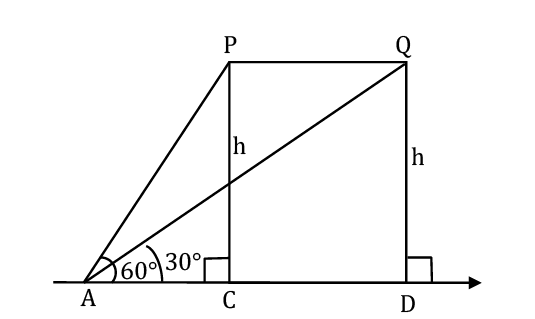v = 432 × 1000/(60×60) m/sec
= 120 m/sec
Distance PQ = v × 20 = 2400 m
In ΔPAC
tan 600 = h/AC
⇒ AC = h/(√3)
In ΔAQD
⇒√3 h = h/√3 + 2400
⇒2h/√3 = 2400
⇒ h = 1200√3 m

Q 17: For the statements p and q, consider the following compound statements:

(a) (~q∧(p→q)) → ~p

(b) ((p∨q))∧~p) → q

Then which of the following statements is correct?

(A) (A) is a tautology but not (B)

(B) (A) and (B) both are not tautologies

(C) (A) and (B) both are tautologies

(D) (B) is a tautology but not (A)(A) is tautology.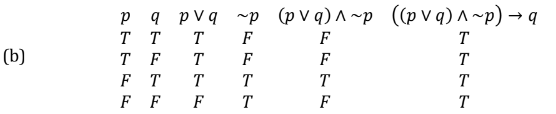(B) is tautology.
∴ (A) and (B) both are tautologies.

Q 18: Let a, b∈R. If the mirror image of the point P(a, 6, 9) with respect to the line (x – 3)/7 = (y – 2)/5 = (z – 1)/(-9) is (20, b, -a, -9), then |a + b| is equal to:

(A) 86

(B) 88

(C) 84

(D) 90

P(a, 6, 9), Q (20, b, -a-9)
Midpoint of PQ=((a+20)/2, (b+6)/2, -a/2) lie on the line.

=> (a + 20 – 6)/14 = (b + 6 – 4)/10 = (-a – 2)/(-18)
=> (a + 14)/14 = (a + 2)/18
=> 18a + 252 = 14a + 28
=> 4a = -224
a = -56
(b + 2)/10 = (a + 2)/18
=> (b + 2)/10 = (-54)/18
=>(b + 2)/10 = -3
=> b = -32
|a + b| = |-56 – 32|
= 88

Q 19: Let f be a twice differentiable function defined on R such that f(0) = 1, f'(0) = 2 and f'(x) ≠ 0 for all x∈R. If |f(x) f'(x) f'(x) f”(x)| = 0, for all x∈R, then the value of f(1) lies in the interval:

(A) (9, 12)

(B) (6, 9)

(C) (3, 6)

(D) (0, 3)

Given f(x) f”(x) – f'(x)2 =0
Let h(x) = f(x)/f'(x)
Then h'(x) = 0
⇒ h(x) = k
⇒ f(x)/f'(x) = k
⇒ f(x) = k f’(x)
⇒ f(0) = k f'(0)
⇒ k = 1/2
Now, f(x) = ½ f'(x)
⇒∫ 2dx = ∫ f'(x)/f(x) dx
⇒ 2x = ln |f(x)| + C
As f(0) = 1 ⇒ C = 0
⇒ 2x = ln |f(x)|
⇒ f(x) = ±e2x
As f(0) = 1 ⇒ f(x) = e2x
∴ f(1) = e2 ≈ 7.38

Q 20: A possible value of tan (¼ sin-1 √63/8) is:

(A) 1/(2√2)

(B) 1/√7

(C) √7 – 1

(D) 2√2 – 1

tan (¼ sin-1 √63/8)
Let sin-1(√63/8) = θ
sin θ = √63/8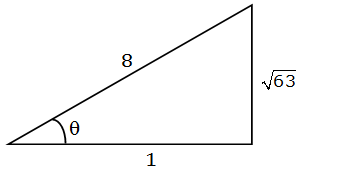cos θ = 1/8
2 cos2(θ/2) – 1 = 1/8
⇒ cos2 θ/2 = 9/16
cos θ/2 = 3/4
⇒(1- tan2 θ/4 )/(1 + tan2 θ/4) = 3/4
tan θ/4 = 1/√7

Section B

Q 1: If the area of the triangle formed by the positive x-axis, the normal and the tangent to the circle (x – 2)2+ (y – 3)2 = 25 at the point (5, 7) is A, then 24A is equal to ___.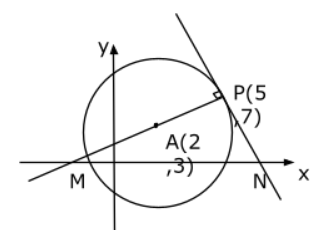Equation of normal at P is
(y – 7) = ((7 – 3)/(5 – 2))(x – 5)
⇒ 3y – 21 = 4x – 20
⇒ 4x – 3y + 1 = 0
⇒ M is (-1/4, 0)
Equation of tangent at P is
(y – 7) = (-¾) (x – 5)
⇒ 4y – 28 = -3x + 15
⇒ 3x + 4y = 43
⇒ N is (43/3, 0)
The question is wrong. The normal cuts at a point on the negative axis.

Q 2: If a + α = 1, b + β = 2 and af(x) + αf(1/x) = bx + β/2, x ≠ 0 then the value of the expression [f(x) + f(1/x) ]/(x + 1/x)

af(x) + αf(1/x) = bx + β/x …(i)
Replace x by 1/x
af(1/x) + αf(x) = b/x + βx …(ii)
(i) + (ii)
(a + α)[f(x) + f(1/x) ]
= (x + 1/x)(b + β)
⇒ ( f(x) + f(1/x))/(x + 1/x)
= 2/1
= 2

Q 3: If the variance of 10 natural numbers 1,1,1,…,1,k is less than 10, then the maximum possible value of k is ________.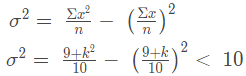⇒ 10(9 + k2) – (81 + k2 + 18k) < 1000
⇒ 90 + 10k2 – k2 – 18k – 81 < 1000
⇒ 9k2– 18k + 9 < 1000
⇒ (k – 1)2 < 1000/9
⇒ k – 1< (10√10)/3
⇒ k < (10√10)/3 + 1
Maximum possible integral value of k is 11.

Q 4: Let a point P be such that its distance from the point (5, 0) is thrice the distance of P from the point (-5, 0). If the locus of the point P is a circle of radius r, then 4r2 is equal to ___.

Let P be (h, k), A(5, 0) and B(-5, 0)
Given PA = 3PB
⇒ PA2 = 9PB2
⇒ (h-5)2 + k2 = 9[(h + 5)2 + k2]
⇒ 8h2 + 8k2 + 100h + 200 = 0
∴ Locus of P is x2 + y2 + (25/2)x + 25 = 0
Centre = (-25/4, 0)
∴ r2 = (-25/4)2 – 25
= 625/16 – 25
= 225/16
∴4r2 = 4×225/16
= 225/4
= 56.25

Q 5: The number of the real roots of the equation (x + 1)2 + |x – 5| = 27/4 is ___.

For x ≥ 5,
(x + 1)2+ (x – 5) = 27/4
⇒ x2 + 3x – 4 = 27/4
⇒ x2 + 3x – 43/4 = 0
⇒ 4x2 + 12x – 43 = 0
x = (-12 ± √(144 + 688))/8
x = (-12 ± √832)/8
= (-12 ± 28.8)/8
= (-3 ± 7.2)/2
= (-3 + 7.2)/2, (-3 – 7.2)/2 (therefore, no solution)
For x < 5,
(x + 1)2– (x – 5) = 27/4
⇒ x2 + x + 6 – 27/4=0
⇒ 4x2 + 4x – 3=0
x = (-4 ± √(16 + 48))/8
x = (-4 ± 8)/8
⇒ x = -12/8, 4/8
∴ 2 real roots.

Q 6: The students S1, S2,… S10 are to be divided into 3 groups A, B, and C such that each group has at least one student and the group C has at most 3 students. Then the total number of possibilities of forming such groups is ___.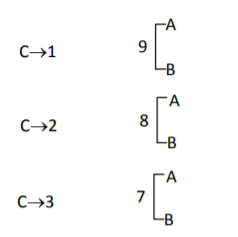Number of ways
= 10C1 [29 – 2] + 10C2 [28– 2] + 10C3 [27 – 2]
= 27 [10C1×4 + 10C2 ×2 + 10C3] – 20 – 90 – 240
= 128 [40 + 90 + 120 ] – 350
= (128 × 250) – 350
= 10 
= 31650

Q 7: For integers n and r, let

The maximum value of k for which the sum

exists, is equal to ____.

(1+x)10 = 10C0 + 10C1x + 10C2x2 + …… + 10C10x10
(1+x)15= 15C0 + 15C1x +15C2x2 + …… + 15Ck-1xk-1 + 15Ck+1xk+1 + …15C15x15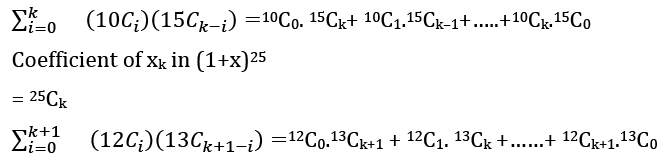Coefficient of xk+1 in (1+x)25
= 25Ck+1
25Ck + 25Ck+1 = 26Ck+1
For maximum value
as per given, k can be as large as possible.

Q 8: Let λ be an integer. If the shortest distance between the lines x – λ = 2y – 1 = -2z and x = y + 2λ = z – λ is √7/2√2, then the value of |λ| is __

(x – λ)/1 = (y – 1/2)/(1/2) = z/(-1/2)
(x – λ)/2 = (y-1/2)/1 = z/(-1) …(1) Point on line = (λ, 1/2, 0)
x/1 = (y + 2λ)/1 = (z – λ)/1 …(2) Point on line = (0, -2λ, λ)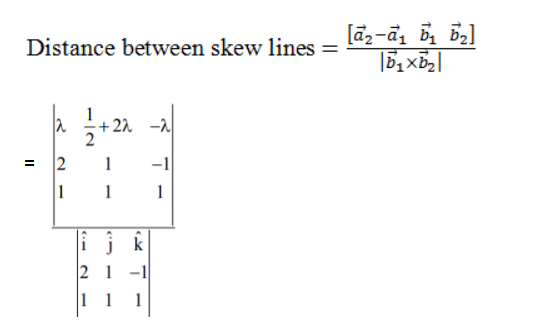= |-5λ – 3/2|/√14
= √7/(2√2) (Given)
⇒ |10λ + 3| = 7
⇒ λ = -1 as λ is an integer.
⇒ |λ| = 1

Q 9: If i = √-1. If [(-1 + i√3)21/(1 – i)24 + (1 + i√3)21/(1 + i)24 ] = k, and n = [|k| ] be the greatest integral part of |k|. Then

is equal to ___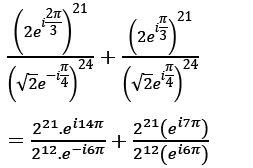= 29 ei(20π) +29 e
= 29 + 29 (-1)
= 0 = k
∴ n = 0
∑(j=0)5 (j + 5)2– ∑(j=0)5 (j + 5)
= [52 + 62 + 72 + 82 + 92 + 102 ] – [5 + 6 + 7 + 8 + 9 + 10]
= [(12+ 22 +… + 102) – (12 + 22+ 32+ 42) ] – [(1 + 2 + 3 + … + 10) – (1 + 2 + 3 + 4) ]
= (385 – 30) -[55 – 10]
= 355 – 45
= 310

Q 10: The sum of first four terms of a geometric progression (G.P.) is 65/12 and the sum of their respective reciprocals is 65/18. If the product of first three terms of the G.P. is 1, and the third term is α then 2α is____

Let terms of GP are a, ar, ar2, ar3
a + ar + ar2 + ar3 = 65/12 …….(1)
1/a + 1/ar + 1/(ar2) + 1/ar3) = 65/18
⇒1/a (r3 + r2 + r + 1)/r3) = 65/18 …….(2)
(1)/(2), we get
a2r3 = 18/12
= 3/2
Also, a3 r3 = 1
⇒ a(3/2) = 1
⇒a = 2/3
(4/9) r3 = 3/2
⇒ r3 = 33/23
⇒ r = 3/2
α = ar2
= 2/3.(3/2)2 = 3/2
∴2α = 3

[/tab]

[/tabs]

×

### Hello!

Click one of our representatives below to chat on WhatsApp or send us an email to info@vidhyarthidarpan.com

×